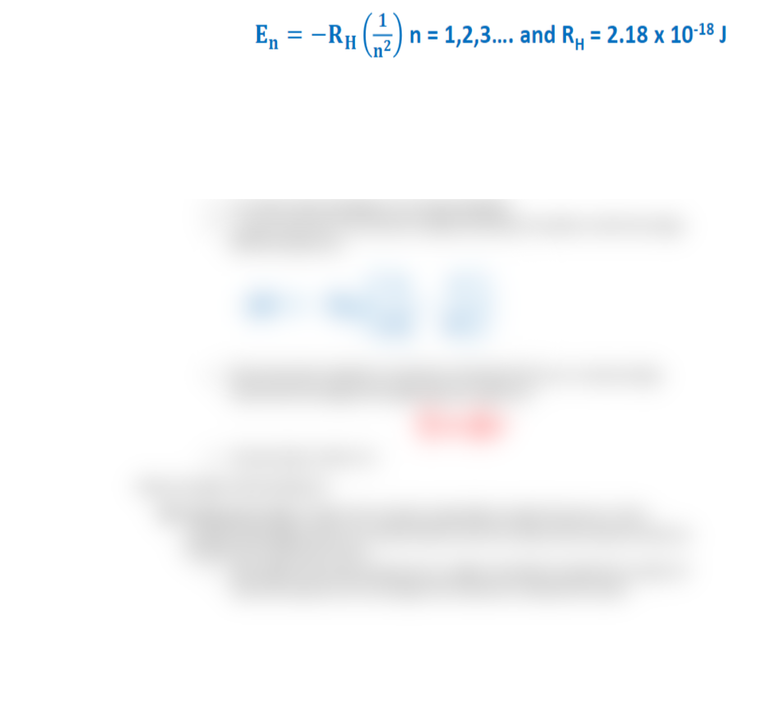Class Notes (1,100,000)
CA (650,000)
UTSC (30,000)
Chemistry (600)
CHMA10H3 (200)
Lecture 11

# CHMA10H3 Lecture 11: Quantum Model of the Atom

Department
Chemistry
Course Code
CHMA10H3
Professor
Marco Zimmer
Lecture
11

This preview shows half of the first page. to view the full 2 pages of the document.CHMA10- Lecture 11 (Jan. 30th)
- The Quantum-Mechanical Model of the Atom
Applying Quantum Mechanics: The Bohr Atom
Bohr took Plank’s idea of quantization and applied it to atomic spectra
o Known fact that molecules and atoms have very specific atomic spectra
(colours)
o Aimed to link spectral data to the underlying structure of a particular compound
or atom by applying quantization to electrons
He developed a math model of the atom with discrete energy levels or shells
o Electrons release or emit light while moving between shells
o Predicted hydrogen atom exactly
Bohr Atom’s Atomic Structure
o Atoms have a number of definite and discrete energy levels (orbitals) in which
an electron may exist without emitting or absorbing electromagnetic radiation
o e- moves in circular orbit about nucleus and its motion is governed by ordinary
laws of mechanics and electrostatics
RESTRICTION: angular momentum of e- is quantized i.e. it can only have
certain discrete values
o An orbital radius increases, the energy increases
o e- may move from one discrete energy level (orbit) to another, with the energy
difference given by:
o Monochromatic radiation is emitted or absorbed when an e- moves energy
levels and the energy of the light photons is given by
E=
hv
o Ground state is when n=1
Nature of Light: Particle Evidence
Photoelectric effect: if light with enough energy (high enough frequency or short
enough wavelength) shines on a metal surface, then the surface emits electrons that are
measure as an electrical current
More light of the same frequency (i.e. higher intensity) increased the number of
electrons ejected, but the energy of the electrons remained the same